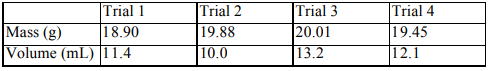# Problem: A student performs an experiment to determine the density of a sugar solution. She performs 4 trials, measuring mass and volume each time. Her data table is shown below.If the correct value for the density of the sugar solution is 1.33 g/mL, which statement best describes her density results?A) Her results are neither precise nor accurate. B) Her results are both accurate and precise. C) Her results are precise, but not accurate. D) Her results are accurate, but not precise.

###### FREE Expert Solution

We’re being asked to identify the statement that represents the density measurements the best.

Recall that:

• Accuracy refers to how close the measurement is to the ‘true/actual value’.

• Precision refers to how close the measurements are to each other.

Additionally, the expression of density is the following:

$\overline{){\mathbf{density}}\left(\mathbf{d}\right){\mathbf{=}}\frac{\mathbf{mass}}{\mathbf{volume}}}$

For this problem, the true value of density is 1.33 g/mL.

86% (181 ratings)###### Problem Details

A student performs an experiment to determine the density of a sugar solution. She performs 4 trials, measuring mass and volume each time. Her data table is shown below.If the correct value for the density of the sugar solution is 1.33 g/mL, which statement best describes her density results?

A) Her results are neither precise nor accurate.

B) Her results are both accurate and precise.

C) Her results are precise, but not accurate.

D) Her results are accurate, but not precise.